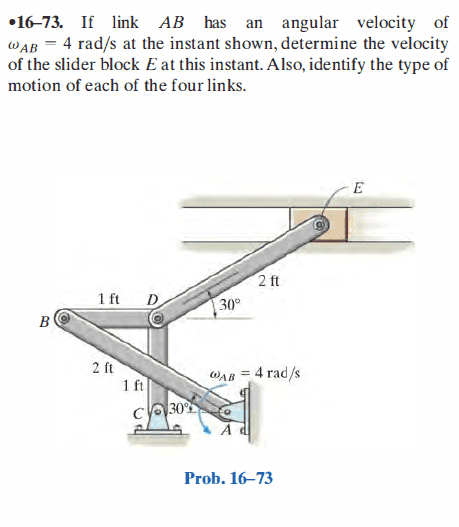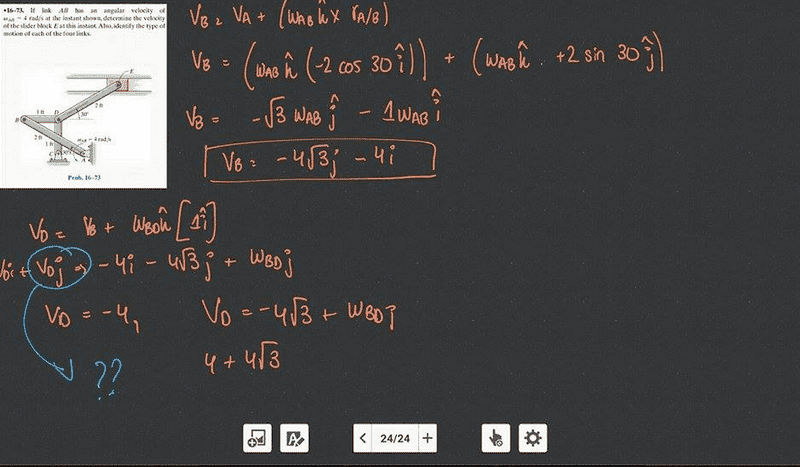# Kinematics using vector analysis

## Homework Statement## Homework Equations

Va = Vb + Va/b
Va = Vb + (wab x ra/b)

## The Attempt at a Solution

OK so here is part of my solution:My velocity for B is correct according to the book. But I'm confused about D. The only problem here is that the velocity of D only has an i component, no j component. How do we know that and why is that? Also, how come the velocity of D isn't zero since it's a fixed point?